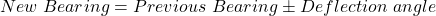# Angle of Deflection – Definition, Formula, & Applications

#### Engineering

Deflection angle is an important concept in several fields including surveying, photogrammetry, and use of radars. Understanding this concept is critical to predicting and modeling the movement of objects in space. In this article, you will learn what a deflection angle is, its formula, and its application to radar use and surveying.

## What is Angle of Deflection

Generally, an angle of deflection refers to the angle between the path of departure of a moving object, and its initial course. Moreover, in fields such as surveying, photogrammetry and gunnery, this definition varies slightly. However, it still centers on the concept of measuring the relative angle between paths. For example, when light passes from one media to another, such as from air through a prism and back to air, the rays refract. Thus, the incident ray changes direction at the air-prism interface. In addition, another change in direction occurs at the prism-air interface by the emergent ray. The angle between the incident and emergent ray is an angle of deflection (α) and is of importance in estimating the refractive index of the prism.

The angle of deflection is key in gunnery. In this field, it refers to the angle between the line of sight to a moving target and the line of sight to the aiming point. In the case of a fighter jet, it uses the value of the angle to lay ahead of a target and provide an accurate time of flight for the weapon to strike its target. Because of the speed of these jets, the angles are often very small, so are normally measured in milliradians.

## Angle of Deflection Formula

The formula for angle of deflection largely depends on the application in question. When light goes through a prism, the angle of deflection (α) is a function of several parameters. Moreover, these parameters include the angle of incidence (ϕ1) and the angle of refraction (θ1) as the ray enters the prism. Also, it depends on the angle of incidence (ϕ2) and the angle of refraction (θ2) as the ray exits the prism.In bearing applications, the measurement of a deflection angle is between a new line and the previous one. If measuring this angle in the clockwise direction, then it has a positive value. When measurement occurs in the anti-clockwise direction, convention requires assigning a negative value.## Application in Surveying

In surveying, a deflection angle refers to a horizontal angle between a preceding line and the following line. In addition, it is critical in the mapping out of the boundaries of a real estate. Although its measurement can be either clockwise or anti-clockwise, its value must lie between 0° and 180°. When using this angle to define a real estate, a reference line, direction of turning, and magnitude of the angular distance are all requirements. Also, some tools that surveyors use in measuring this angle include a theodolite, compass, and sextant. Typically, on a survey plan, when the direction of rotation of the angle is to the right, the letter ‘R’ indicates it, while ‘L’ indicates a measurement to the left, as the diagram below shows.